Courses

# Test: Coordination Compounds 1 - From Past 28 Years Questions

## 37 Questions MCQ Test Chemistry 28 Years Past year papers for NEET/AIPMT Class 12 | Test: Coordination Compounds 1 - From Past 28 Years Questions

Description
This mock test of Test: Coordination Compounds 1 - From Past 28 Years Questions for NEET helps you for every NEET entrance exam. This contains 37 Multiple Choice Questions for NEET Test: Coordination Compounds 1 - From Past 28 Years Questions (mcq) to study with solutions a complete question bank. The solved questions answers in this Test: Coordination Compounds 1 - From Past 28 Years Questions quiz give you a good mix of easy questions and tough questions. NEET students definitely take this Test: Coordination Compounds 1 - From Past 28 Years Questions exercise for a better result in the exam. You can find other Test: Coordination Compounds 1 - From Past 28 Years Questions extra questions, long questions & short questions for NEET on EduRev as well by searching above.
QUESTION: 1

### Which of the following is considered to be an anticancer species ?

Solution:

Diaminodichloroplatinum (II) commonly known as  - platin is found to have anticancer property.

QUESTION: 2

### Which of the following coordination compounds would exhibit optical isomerism? 

Solution:

The optical isomers are pair of molecules which are non super imposable mirror images of each other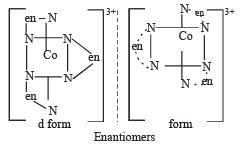The two optically active isomers are collectivity called enantiomers.

QUESTION: 3

### Among [ Ni(CO)4],[ Ni(CN) 4]2- ,[ NiCl4]2- species, the hybridization states of the Ni atom are, respectively(At. No. of Ni = 28) 

Solution:

28 Ni0 :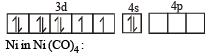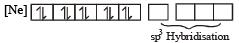Remember CO is a strong ligand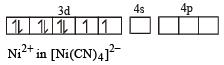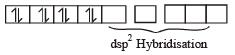Remember CN is also a strong ligand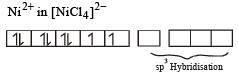Cl is a weak ligand, hence no pairing of electrons takes place.

QUESTION: 4

CN– is a strong field ligand. This is due to the fact that 

Solution:

CN is astrong fieldlig and as it is a psuedohalide ion. These ions are strong coordinating ligands and hence have the tendency to form σ-bond (from the pseudo halide to the metal) and π-bond. (from the metal to pseudo halide)

QUESTION: 5

Considering H2O as a weak field ligand, the number of unpaired electrons in [Mn(H2O)6]2+ will be (At. no. of Mn = 25) 

Solution:

Since H2O is a weak ligand, it will not cause pairing of electrons in the metal ion Mn2+.
Thus electronic configuration of the metal (Mn2+) in the complex will be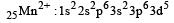i.e s unpaired electrons.

QUESTION: 6

Which of the following does not have a metalcarbon bond? 

Solution:

Triethoxyaluminium has no Al – C linkage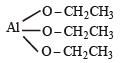QUESTION: 7

Which one of the following is an inner or bital complex as well as diamagnetic in behaviour? (Atomic number: Zn = 30, Cr = 24, Co = 27, Ni = 28) [2 00 5]

Solution: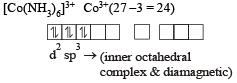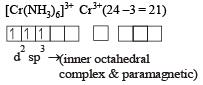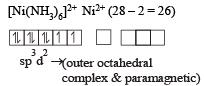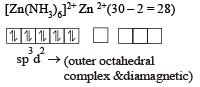QUESTION: 8

Which one of the following is expected to exhibit optical isomerism? (en = eth ylen ediamine) [2 00 5]

Solution: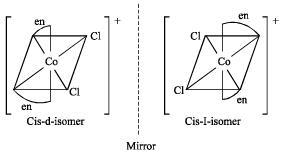Transform of [M(AA)2 a2] does not shows optical isomerism.

QUESTION: 9

[Co(NH3)4 (NO2)2] Cl exhibits 

Solution:

The given compound may have linkage isomerism due to presence of NO2 group which may be in the form –NO2 or –ONO.
It may have ionisation isomerism due to presence of two ionisable group –NO2 & – Cl. It may have geometrical isomerism in the form of form as follows :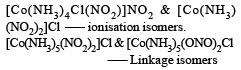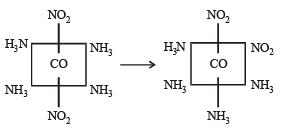Geometrical isomers

QUESTION: 10

[Cr(H2O)6]Cl3 (at no. of Cr = 24) has a magnetic moment of 3.83 B. M. The correct distribution of 3d electrons in the Chromium of the complex is

Solution: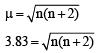on solving n = 3

as per magnetic moment, it has three unpaired electron.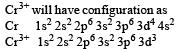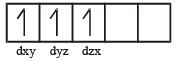So      3dxy' 3dyz' 3dxz'

QUESTION: 11

Which of the following will give a pair of enantiomorphs? 

Solution:

Non superimposable mirror images are called optical isomers and may be described as “chiral’. They are also called enantiomers and rotate plane polarised light in opposite directions.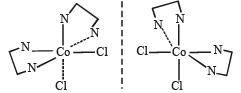QUESTION: 12

The d electron configurations of Cr2+, Mn2+, Fe2+ and Ni2+ are 3d4, 3d5, 3d6 and 3d8 respectively. Which one of the following aqua complexes will exhibit the minimum paramagnetic behaviour?            (At. No. Cr = 24, Mn = 25, Fe = 26, Ni = 28)

Solution:

Lesser is the number of unpaired electrons smaller will be the paramagnetic behaviour.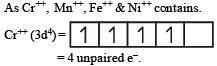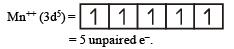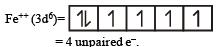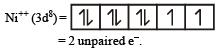As Ni++ has minimum no. of unpaired e thus this is least paramagnetic.

QUESTION: 13

Which of the following complexes exhibits the highest paramagnetic behaviour ?        where gly = glycine, en = ethylenediamine and bpy = bipyridyl moities) (At.nosTi = 22, V = 23, Fe = 26, Co = 27)

Solution:

More the number of unpaired electrons present in a complex more is its paramagnetic behaviour.
To find unpaired electrons let us calculate the oxidation states of elements in each complex and then write the electronic configuration for that oxidation state to find the number of unpaired electrons in it.
We find that in their complexes V, Fe, Co and Ti are in + 3, + 2, + 5, and + 3 oxidation states respectively. In these state the number of unpaired electrons present in them are 2, 4, 4 and 1 respectively. Since the maximum number of unpaired electrons are in cobalt complex so it has highest paramagnetic behaviour i.e. choice (c) is correct answer.

QUESTION: 14

In which of the following coordination entities the magnitude Δ0 (CFSE in octahedral field) will be maximum? 

Solution:

In octahedral field the crystal field splitting of d- orbitals of a metal ion depends upon the field produced by the ligands.
In general ligands can be arranged in a series in the order of increasing fields and splittings which they produce around a central metal ion. A portion of the series is given below. cyanide > ethylene - diamine > ammonia > pyridine>thiocyanate > water> fluoride > oxalate > hydroxide> chloride> bromide > iodide.
Out of the given ligands water, ammonia, cyanide and oxalate, we can find from the above series of ligands that the maximum splitting will occur in case of cyanide (CN) i.e. the magnitude of Δ0 will be maximum in case of [Co(CN)6]3+.

QUESTION: 15

Which of the following does not show optical isomerism? 

Solution:

The octahedral coordination compounds of the type MA3B3 exhibit fac-mer isomerism.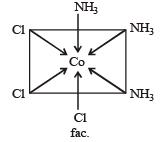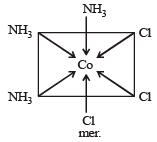QUESTION: 16

Which of the following complex ions is expected to absorb visible light?              (At. no. Zn = 30, Sc = 21, Ti = 22, Cr = 24)

Solution:

Since Cr3+ in the complex has unpaired electrons in the d orbital, hence will be coloured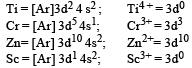QUESTION: 17

Which of the following complex ion is not expected to absorb visible light ? 

Solution: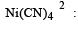Number of unpaired electrons = 0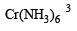: Number of unpaired electrons = 3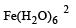: Number of unpaired electrons = 4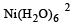: Number of unpaired electrons = 2

QUESTION: 18

Crystal field stabilization energy for high spin 4 octahedral complex is: 

Solution:

4 in high spin octahedral complex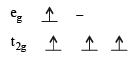CFSE = [0.6 × 1] + [–0.4 × 3] = – 0.6 Δ0

QUESTION: 19

The existence of two different coloured complexes with the composition of [Co(NH3)4 Cl2]+ is due to : 

Solution: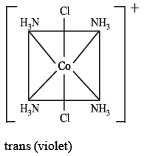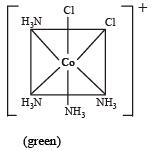QUESTION: 20

Which one of the following complexes is not expected to exhibit isomerism?              

Solution: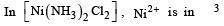hybridisation, thus tetrahedral in shape. Hence the four ligands are not different to exhibit optical isomerism.

∴      Correct choice : (d)

QUESTION: 21

Of the following complex ions, which is diamagnetic in nature ? 

Solution: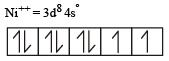Since, the coordination number of Ni in this complex is 4, the configuration of Ni++ at first sight shows that the complex is paramagnetic with two unpaired electron. However, experiments show that the complex is diamagnetic. This is possible when the 3d electrons rearrange against the Hund’s rule as shown below. This is in accordance with  the fact that the ligand involved here is strong i.e., CN ion.

Ni++ (after rearrangement)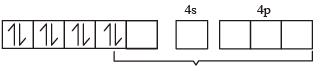Hence, now dsp2 hybridization involving one 3d, one 4s and two 4p orbitals, takes place leading to four dsp2 hybrid orbitals, each of which accepts four electron pairs from CN ion forming [Ni (CN)4]2– ion.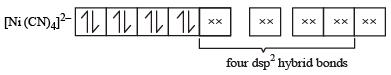Thus, the complex is diamagnetic as it has no unpaired electron.

QUESTION: 22

Th e complexes [Co(NH3)6] [Cr (CN)6] and [Cr(NH3)6] [Co(CN)6] are the examples of which type of isomerism? 

Solution:

Coordination isomerism occurs when cationic and anionic complexes of different metal ions are present in a salt. Interchange of ligand between the complexes give isomers e.g. [Co (NH3)6] [Cr (CN)6]  is an isomer of [Co (CN)6] [Cr (NH3)6]

QUESTION: 23

The complex, [Pt(Py)(NH3)BrCl] will have how many geometrical isomers ? 

Solution:

Complexes of the type MABCD may exist in three isomeric forms.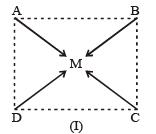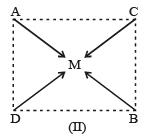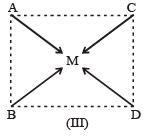Similarly, [Pt (Py) (NH3) BrCl] may exist in three isomeric form in which M = Pt A = Py,  B = NH3,  C = Br,  D = Cl.

QUESTION: 24

The d-electron configurations of Cr 2+, Mn2+, Fe2+ and Co2+ are d4, d5, d6 and d7, respectively.Which one of the following will exhibit minimum paramagnetic behaviour?                  (At, nos. Cr = 24, Mn = 25, Fe = 26, Co = 27)

Solution: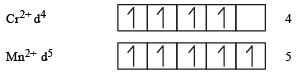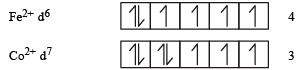Minimum paramagnetic behaviour = [Co (H2O)6]2+

QUESTION: 25

Which of the following carbonyls will have the strongest C – O bond ? [2011 M]

Solution:

As + ve charge on the central metal atom increases, the less readily the metal can donate electron density into the π* orbitals of CO ligand to weaken the C – O bond.
Hence, the C – O bond would be strongest in Mn(CO)6+.

QUESTION: 26

Which of the following complex compounds will exhibit highest paramagnetic behaviour? [2011M] (At. No. : Ti = 22, Cr = 24, Co = 27, Zn = 30)

Solution:

(a) [Ti(NH3)6]3+ : 3d1 configuration and thus has one unpaired electron. (b) [Cr(NH3)6]3+ : In this complex Cr is in +3 oxidation state.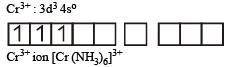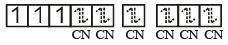Thus, the complex is paramagnetic.

(c) [Co(NH3)6]3+ : In this complex are cobalt ion is in + 3 oxidation state with 3d6 configuration.
Co3+,[Ar]3d6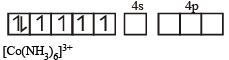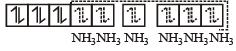(inner orbital or d2sp3 hybrid orbital low spin complex) diamagnetic

(d) In this complex Zn exists as

Zn++ ion

Zn++ ion : 3d10 4s0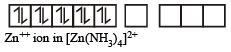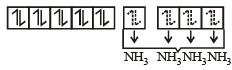Due to presence of paired electrons complex is diamagnetic in nature.

QUESTION: 27

Which one of the following is an outer orbital complex and exhibits paramagnetic behaviour ? 

Solution:

[Ni(NH3)6]2+

Ni2+ = 3d8, according to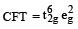therefore, hybridisation is sp3d2 & complex is paramagnetic.

QUESTION: 28

Red precipitate is obtained when ethanol solution of dimethylglyoxime is added to ammoniacal Ni(II). Which of the following statements is not true ? [2012 M]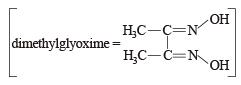Solution:

Nickel ions are frequently detected by the formation of red precipitate of the complex of nickel dimethylglyoxime, when heated with dimethylglyoxime.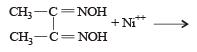Dimethylglyoxime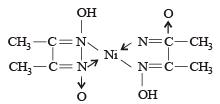Nickel dimethylglyoxime

QUESTION: 29

Low spin complex of d6-cation in an octahedral field will have the following energy : [2012 M]    (Δ0= Crystal Field Splitting Energy in an octahedral field, P = Electron pairing energy)

Solution: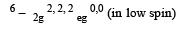C.F.S.E  =  – 0.4 × 6Δ0 + 3P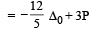QUESTION: 30

A magnetic moment of 1.73 BM will be shown by one among the following : [NEET 2013]

Solution:

[Cu(NH3)4]2+ hybridisation 2 Cu+2 – 3 9 has one unpaired e-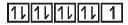So magnetic moment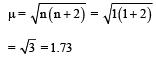QUESTION: 31

An excess of AgNO3 is added to 100 mL of a 0.01 M solution of dichlorotetraaquachromium (iii) chloride. The number of moles of AgCl precipitated would be : [NEET 2013]

Solution: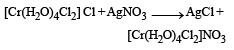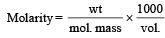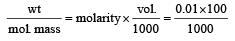QUESTION: 32

The correct IUPAC name for [CrF2(en)2]Cl is: [NEET Kar. 2013]

Solution:

IUPAC name of [CrF2 (en)2]Cl is Difluoridobis(ethylenediamine)chromium (III) chloride.

QUESTION: 33

Crystal field splitting energy for high spin 4 octahedral complex is:    [NEET Kar. 2013]

Solution:

CFSE = (– 0.4x + 0.6y) Δ0

where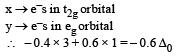QUESTION: 34

Which is diamagnetic?         [NEET Kar. 2013]

Solution: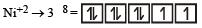CN is a strong ligand and causes pairing of 3 electrons of Ni2+.

∴           It is diamagnetic.

QUESTION: 35

In a particular isomer of  [Co(NH3)4Cl2]0, the Cl-Co-Cl angle is 90°, the isomer is known as     [NEET Kar. 2013]

Solution:

The correct answer is Option C.
The Cl−Co−Cl bond is at right angle.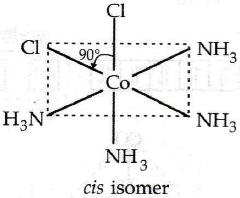QUESTION: 36

The anion of acetylacetone (a cac) for ms Co(acac)3 chelate with Co3+. The rings of the chelate are [NEET Kar. 2013]

Solution:

Acetylacetone forms six membered stable ring complexes.

QUESTION: 37

Which among the following is a paramagnetic complex? [NEET Kar. 2013]

Solution: